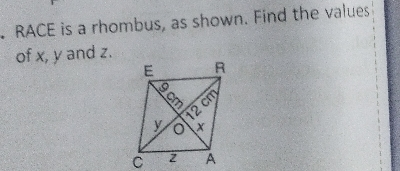"
">

# $\mathrm{RACE}$ is a rhombus, as shown. Find the valuesof $x, y$ and $z$."

Given: $RACE$ is a rhombus, as shown in the figure.

To do: To find $x,\ y$ and $z$.

Solution:$\because RACE$ is a rhombus.

$\therefore$ Diagonals $RC$ and $AE$ bisects each other at $90^o$.

$\Rightarrow OC=OR$ and $OA=OE$

$\Rightarrow y=12\ cm$ and $x=9\ cm$

In right angled $\vartriangle AOC$, on using Pythagoras theorem,

$z^2=x^2+y^2$

$\Rightarrow z^2=9^2+12^2$

$\Rightarrow z^2=81+144$

$\Rightarrow z^2=225$

$\Rightarrow z=\sqrt{225}$

$\Rightarrow z=15\ cm$

Thus, $x=9\ cm, y=12\ cm$ and $z=15\ cm$.

Updated on: 10-Oct-2022

56 Views